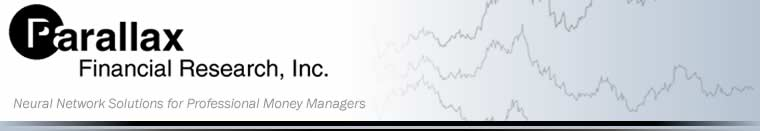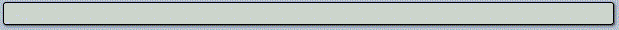Our Technology | Feedback | Scale Invariance | Spatial Probability | Neural Networks | Validation

## Scale Invariance & Chaos Theory

R.N. Elliott observed that financial series appear to have the same "look" on many different time scales. Elliott published what he called the "Wave Principle in 1938. His modeled financial series is comprised of zigzags that are nested within each other at smaller and smaller scales. Market technicians still make wide use of his work.

####Scientists now understand this phenomenon as scale invariance. Scale invariance (also known as self-similarity) is defined as a feature of objects or laws that do not change if length scales are multiplied by a common factor. Its presence means that the financial series has been produced by feedback and is governed by the rules and the predicted behavior of chaos theory. Chaos theory is the science of feedback systems. As financial scientists, we seek to understand which market characteristics are predicted by chaos theory and then build a set of predictive tools that capture what is theoretically possible. In many cases, we find inspiration from existing technical analysis methods.

####Financial series are what Benoit Mandelbrot called a fractal. Not quite like a fern or a snowflake, which have a constant fractal dimension, but a statistical fractal, with imperfections and varying dimension. Financial series exhibit approximate fractal-like scale invariance only sporadically. Our research, as well as that of fellow geophysicist Didier Sornette, confirms that financial series exhibit "discrete scale invariance" near major trend changes.